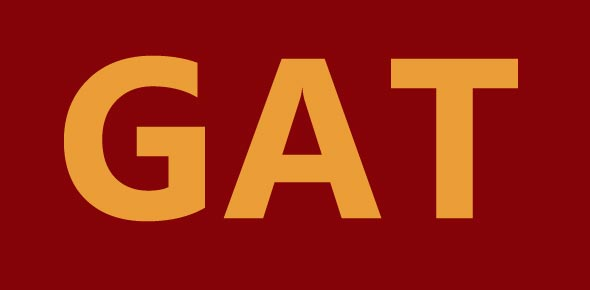113 Questions | Total Attempts: 675SettingsOverview of formulas, arithmetic, and idioms to memorize for the GMAT.

• 1.
12.5% = what fraction?
• 2.
16.66% = what fraction?
• 3.
37.5% = what fraction?
• 4.
62.5% = what fraction?
• 5.
66.66% = what fraction?
• 6.
83.33% = what fraction?
• 7.
87.5% = what fraction?
• 8.
1/8 = what percent?
• 9.
1/6 = what percent?
• 10.
3/8 = what percent?
• 11.
5/8 = what percent?
• 12.
5/6 = what percent?
• 13.
7/8 = what percent?
• 14.
112=?
• 15.
122=?
• 16.
132=?
• 17.
142=?
• 18.
152=?
• 19.
43=?
• 20.
53=?
• 21.
63=?
• 22.
73=?
• 23.
83=?
• 24.
93=?
• 25.
√2 ≈ ?
Related TopicsBack to top